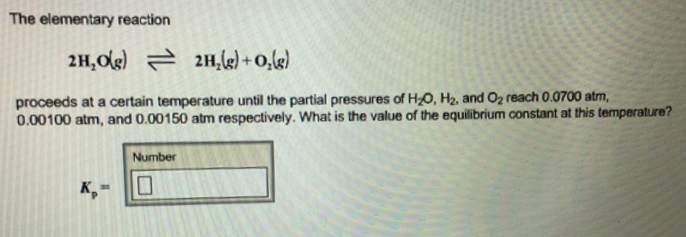# Problem: The elementary reaction 2H2O(g) ⇌ 2H2(g) + O2(g) proceeds at a certain temperature until the partial pressures of H2O, H2, and O2 reach 0.0700 atm, 0.00100 atm, and 0.00150 atm respectively. What is the value of the equilibrium constant at this temperature?

🤓 Based on our data, we think this question is relevant for Professor Wallace's class at LEHMAN-CUNY.

###### FREE Expert Solution###### Problem Details

The elementary reaction

2H2O(g) ⇌ 2H2(g) + O2(g)

proceeds at a certain temperature until the partial pressures of H2O, H2, and O2 reach 0.0700 atm, 0.00100 atm, and 0.00150 atm respectively. What is the value of the equilibrium constant at this temperature?